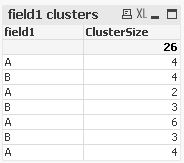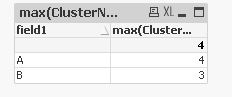# QlikView Scripting

Discussion Board for collaboration on QlikView Scripting.

Announcements
Modernize Your QlikView Deployment webinar, Nov. 3rd. REGISTER
cancel
Showing results for
Did you mean:
HighlightedNot applicable

## count until value changes (in diagram)

hello and a happy new year to everyone

i've got a question about a counting function:

how can i count the rows until the value in one column changes.

ex:

A

A

A

A

A

A

B

B

B

A

A

A

A

i want to count all "A's" before the value changes to "B"

1 Solution

Accepted Solutions
HighlightedMVP

Script:

Table:

If(Peek('Example') = Example, Peek('Same'), RangeSum(1, Peek('Same'))) as Same;

Example

A

A

A

A

B

B

B

B

A

A

B

B

B

];

Straight Table

Dimension: Example and Same

Expression: Count(Example)10 Replies
HighlightedMVP

Where are you wanting to count this? Script or front end? And you only want to count As? and not Bs

HighlightedNot applicable

i think i will count on diagram. but it can be in script too.

yes, i have to know how much rows are there before the first B is in. I want to count the Bs as well, but only the uninterrupted Bs.

Example

A

A

A

A

B

B

B

B

A

A

B

B

B

In this example i would like to count the first As, then the First Bs, then de second As and the second Bs - ist that even possible?

HighlightedMVP

Script:

Table:

If(Peek('Example') = Example, Peek('Same'), RangeSum(1, Peek('Same'))) as Same;

Example

A

A

A

A

B

B

B

B

A

A

B

B

B

];

Straight Table

Dimension: Example and Same

Expression: Count(Example)HighlightedMVP

Hi,```table1:
AutoNumber(ID,ClusterTotNum) as field1Seq,
AutoNumber(ClusterTotNum,field1) as ClusterNum;
*,
Alt(Peek(ClusterTotNum),0)-(Previous(field1)<>field1) as ClusterTotNum
Inline [
field1
A
A
A
A
B
B
B
B
A
A
B
B
B
A
A
A
A
A
A
B
B
B
A
A
A
A
];

Join
Count(field1) as ClusterSize
Resident table1
Group By ClusterTotNum;
```
HighlightedNot applicable

thank you! this will help me!

HighlightedNot applicable

thank you! this will help me!

HighlightedMVP

you're welcome.

If you like, you can mark this solution helpful.

thanks

regards

Marco

HighlightedNot applicable

what if I want to count the number of clusters with the same label, output example:

A: 4

B: 3

Thanks!

HighlightedNot applicable

I figured it out by using the expression as max(ClusterNum):This is very useful to count the number of clusters.